## Get the Current Workbook Name

This post explains how to get the current workbook name in excel.

## Get the Current Workbook Name

In excel, the CELL function can get the information of the current worksheet, it will return the full file name and its path. If you want to get the name of the current workbook only, you can use a combination of the MID function, the CELL function and the FIND Function. Just refer to the below generic formula:

`=MID(CELL("filename",B1),FIND("[",CELL("filename",B1))+1,FIND("]",CELL("filename",B1))- FIND("[",CELL("filename",B1))-1)`

Let’s see how the above formula works:

The MID function will extract a sub-string from a text string at the position that you specify. It contains three arguments in MID function.

The first argument is the returned results of the first CELL function. The full workbook name and its path will be returned from the below CELL function.

`=CELL("filename",B1)`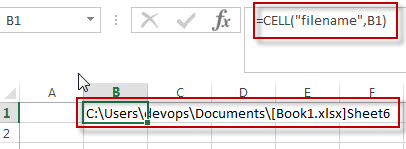The first FIND function will locate the position of the first bracket along with the workbook name. It is the second arguments as the MID function.

`=FIND("[",CELL("filename",B1))`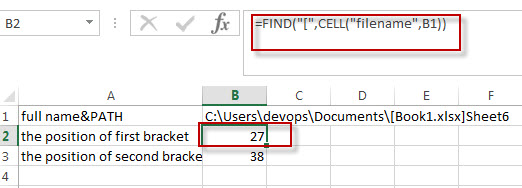The second FIND function will return the position of the second bracket right of the workbook name.

`=FIND("]",CELL("filename",B1))`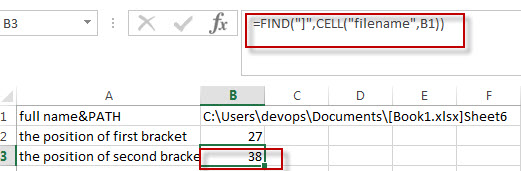The third argument of the MID function is the length of the substring that you want to extract. In other words, you need to get the length of the workbook name. Using the below formula:

`=FIND("]",CELL("filename",B1))- FIND("[",CELL("filename",B1))-1`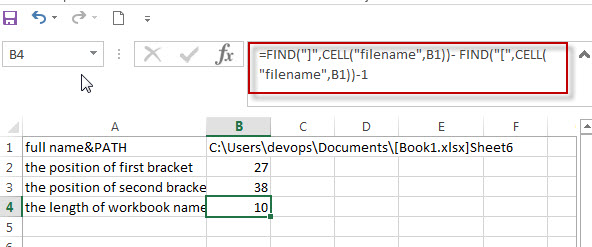Last, we can run the following excel formula to get the current workbook name:

`=MID(CELL("filename",B1),FIND("[",CELL("filename",B1))+1,FIND("]",CELL("filename",B1))- FIND("[",CELL("filename",B1))-1`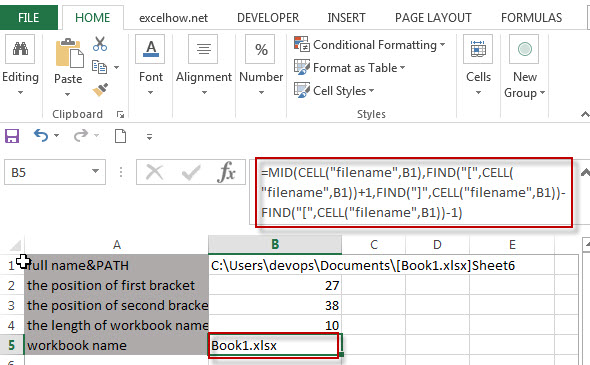### Related Functions

• Excel Find function
The Excel FIND function returns the position of the first text string (substring) from the first character of the second text string.The FIND function is a build-in function in Microsoft Excel and it is categorized as a Text Function.The syntax of the FIND function is as below:= FIND  (find_text, within_text,[start_num])…
• Excel MID function
The Excel MID function returns a substring from a text string at the position that you specify.The syntax of the MID function is as below:= MID (text, start_num, num_chars)…
• Excel CELL function
The Excel CELL function returns information about the formatting, location, size, or contents of a cell.The syntax of the CELL function is as below:= CELL (info_type,[reference])…

## Split Text and Numbers in Excel

This post explains how to split text and numbers in excel, or how to extract the text characters from another strings with text and numbers combined.

## Split Text and Numbers

If you want to split text and numbers, you can run the following excel formula that use the MIN function, FIND function and the LEN function within the LEFT function in excel. And if you want to extract only numbers within string, then you also need to RIGHT function to combine with the above functions.

`=LEFT(MIN(FIND({0,1,2,3,4,5,6,7,8,9},B1&"0123456789")))`

To split the text and numbers, the key is to get the position of the first digit within the Cell B1, once the position of the first number is located, you can use the LEFT function or RIGHT function to extract text characters or just numbers.

Let’s see how the above formula works as below:

To get the position of the first number with the cell B1, and you can use the following formula:

`=MIN(FIND({0,1,2,3,4,5,6,7,8,9},B1&"0123456789"))`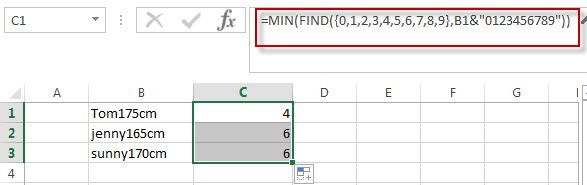The position of the first number is returned from the above Excel formula.

The Find function will locate the starting position of the first number within the cell B1. For the second argument in FIND function, the array constant is used, and the find function will use this array constant to perform a separate search for each number in the array.  And then the returned value of the FIND function also will be an array that contains the position of all numbers in string. The MIN function will use the returned array value of the FIND function to get the minimal value, it is the position of the first number within the Cell B1.

To extract text characters within the Cell B1, using the following formula:

`=LEFT(B1, MIN(FIND({0,1,2,3,4,5,6,7,8,9},B1&"0123456789"))-1)`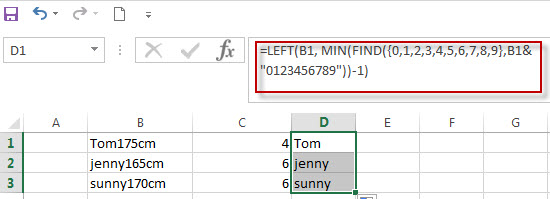To extract numbers within the cell B1, using the following formula:

`=RIGHT(B1,LEN(B1)- MIN(FIND({0,1,2,3,4,5,6,7,8,9},B1&"0123456789"))+1)`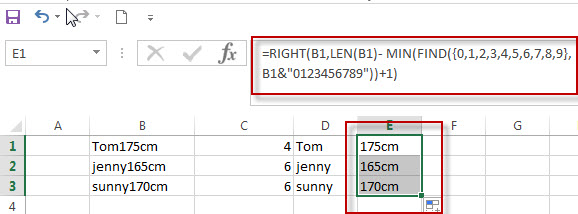The above formula is only works for the string of “text+number” pattern.

## Split String with Numbers and Text combined (Number+Text)

If you need to split the string that the text characters appears after number, the key is to get the length of all numbers left to of the text characters in the Cell B1.  Just using the following formula:

`= SUM(LEN(B1) - LEN(SUBSTITUTE(B1, {0,1,2,3,4,5,6,7,8,9}, "")))`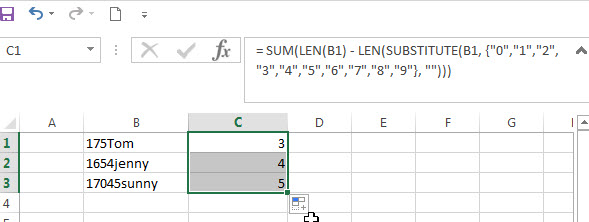In the above formula, the SUBSTITUTE function will use the array constant {0,1,2,3,4,5,6,7,8,9}, it will perform a separate replace for each value in the array constant with new_text (empty string). Then it will return another array with 10 values.  And the second LEN function will use the returned array values of SUBSTITUTE function to get the length of each string. The First LEN function will return the length of the cell B1. The SUM function will calculate the length of numbers with in Cell B1.

To extract numbers within cell B1, using the following formula:

`=LEFT(B1,SUM(LEN(B1)-LEN(SUBSTITUTE(B1,{0,1,2,3,4,5,6,7,8,9},""))))`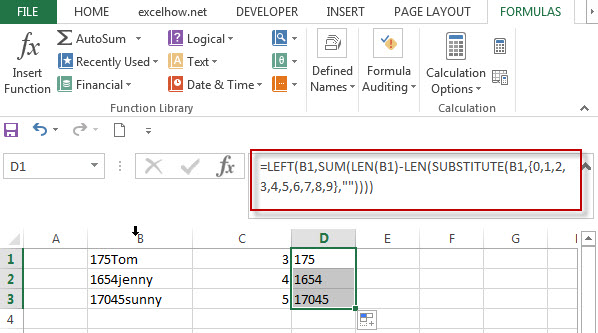To extract text within Cell B1, using the following formula:

`=RIGHT(B1,LEN(B1)- SUM(LEN(B1)-LEN(SUBSTITUTE(B1, {0,1,2,3,4,5,6,7,8,9}, ""))))`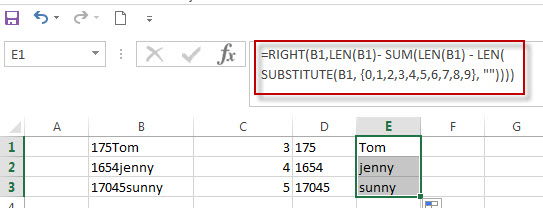### Related Formulas

• Split Text String by Specified Character in Excel
you should use the Left function in combination with FIND function to split the text string in excel. And we can refer to the below formula that will find the position of “-“in Cell B1 and extract all the characters to the left of the dash character “-“.=LEFT(B1,FIND(“-“,B1,1)-1).…
• Split Multiple Lines from a Cell into Rows
If you have multiple lines in a cell and each line is separated by line break character or press “alt + enter” key while you entering the text string into cells, and you need to extract the multiple lines into the separated rows or columns, you can use a combination with the TRIM function, the MID function, the SUBSTITUTE function, the REPT function, the LEN function to create a complex excel formula..…
• Split Text String by Line Break in Excel
When you want to split text string by line break in excel, you should use a combination of the LEFT, RIGHT, CHAR, LEN and FIND functions. The CHAR (10) function will return the line break character, the FIND function will use the returned value of the CHAR function as the first argument to locate the position of the line break character within the cell B1.…
• Split Text and Numbers
If you want to split text and numbers, you can run the following excel formula that use the MIN function, FIND function and the LEN function within the LEFT function in excel. And if you want to extract only numbers within string, then you also need to RIGHT function to combine with the above functions..…

### Related Functions

• Excel Find function
The Excel FIND function returns the position of the first text string (substring) from the first character of the second text string.The FIND function is a build-in function in Microsoft Excel and it is categorized as a Text Function.The syntax of the FIND function is as below:= FIND  (find_text, within_text,[start_num])…
• Excel SUBSTITUTE function
The Excel SUBSTITUTE function replaces a new text string for an old text string in a text string.The syntax of the SUBSTITUTE function is as below:= SUBSTITUTE  (text, old_text, new_text,[instance_num])…
• Excel LEFT function
The Excel LEFT function returns a substring (a specified number of the characters) from a text string, starting from the leftmost character.The LEFT function is a build-in function in Microsoft Excel and it is categorized as a Text Function.The syntax of the LEFT function is as below:= LEFT(text,[num_chars])…
• Excel LEN function
The Excel LEN function returns the length of a text string (the number of characters in a text string).The LEN function is a build-in function in Microsoft Excel and it is categorized as a Text Function.The syntax of the LEN function is as below:= LEN(text)…
• Excel MIN function
The Excel MIN function returns the smallest numeric value from the numbers that you provided. Or returns the smallest value in the array.The syntax of the MIN function is as below:= MIN(num1,[num2,…numn]
• Excel RIGHT function
The Excel RIGHT function returns a substring (a specified number of the characters) from a text string, starting from the rightmost character.The syntax of the RIGHT function is as below:= RIGHT (text,[num_chars])…
• Excel SUM function
The Excel SUM function will adds all numbers in a range of cells and returns the sum of these values. You can add individual values, cell references or ranges in excel.The syntax of the SUM function is as below:= SUM(number1,[number2],…)…

## Split Text String by Specified Character in Excel

This post explains how to split text string by a specified character (such as: comma, space, dash, slash, etc.) using FIND function within other functions in excel. And you will learn that how to extract part of characters to the left of a specified character in one cell.

## Split Text String by Specified Character

When you want to split text string by a specified character, such as:  dash character, the most important thing is that how to get the position of the specified character (dash “-”) within the text string in one cell. So you can use the FIND function or SEARCH Function to get the position of the searched character within another string. Once you got the position number of the specified character, you can use the LEFT function to extract all the characters to the left of dash character (“-“).

So you should use the Left function in combination with FIND function to split the text string in excel. And we can refer to the below formula that will find the position of “-“in Cell B1 and extract all the characters to the left of the dash character “-“.

`=LEFT(B1,FIND("-",B1,1)-1)`

Suppose you have a list of student’s height in column B and you want to extract only the name part. You can use the above excel formula in Cell C1.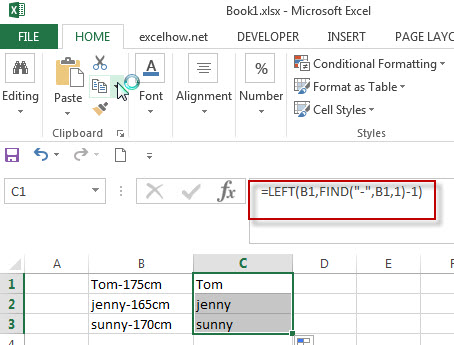In the above example, the FIND function will locate the position of the first dash character (“-“) in Cell B1, and then then LEFT function will use the returned position number of FIND function as the second argument to extract all the characters left (student’s name) to it.

The returned value of FIND function need to subtract 1, as we need to exclude the dash character “-“from the result of the LEFT function.

Of course, if you want to extract all characters to the right of dash character within the text string in Cell B1, you can use a combination of the RIGHT, LEN and FIND functions in excel.  Enter the below excel formula in Cell D1:

`=RIGHT(B1, LEN(B1)-FIND("-",B1))`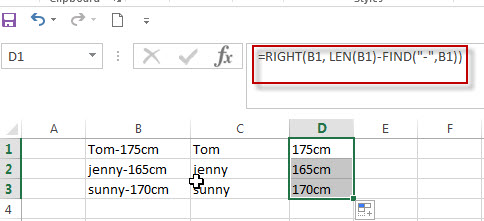In the above example, the FIND function will locate the position of the dash character in Cell B1. And the LEN Function will get the length of the text string in Cell B1. Then subtract the result of FIND function from the returned value of LEN function, so that it will get the number of characters from the rightmost character in Cell B1.

### Related Formulas

• Split Multiple Lines from a Cell into Rows
If you have multiple lines in a cell and each line is separated by line break character or press “alt + enter” key while you entering the text string into cells, and you need to extract the multiple lines into the separated rows or columns, you can use a combination with the TRIM function, the MID function, the SUBSTITUTE function, the REPT function, the LEN function to create a complex excel formula..…
• Split Text String to an Array
If you want to convert a text string into an array that split each character in text as an element, you can use an excel formula to achieve this result. the below will guide you how to use a combination of the MID function, the ROW function, the INDIRECT function and the LEN function to split a string…
• Split Text String by Line Break in Excel
When you want to split text string by line break in excel, you should use a combination of the LEFT, RIGHT, CHAR, LEN and FIND functions. The CHAR (10) function will return the line break character, the FIND function will use the returned value of the CHAR function as the first argument to locate the position of the line break character within the cell B1.…
• Split Text and Numbers
If you want to split text and numbers, you can run the following excel formula that use the MIN function, FIND function and the LEN function within the LEFT function in excel. And if you want to extract only numbers within string, then you also need to RIGHT function to combine with the above functions..…

### Related Functions

• Excel Find function
The Excel FIND function returns the position of the first text string (substring) from the first character of the second text string.The FIND function is a build-in function in Microsoft Excel and it is categorized as a Text Function.The syntax of the FIND function is as below:= FIND  (find_text, within_text,[start_num])…
• Excel SEARCH function
The Excel SEARCH function returns the number of the starting location of a substring in a text string.The syntax of the SEARCH function is as below:= SEARCH  (find_text, within_text,[start_num])…
• Excel LEFT function
The Excel LEFT function returns a substring (a specified number of the characters) from a text string, starting from the leftmost character.The LEFT function is a build-in function in Microsoft Excel and it is categorized as a Text Function.The syntax of the LEFT function is as below:= LEFT(text,[num_chars])…
• Excel LEN function
The Excel LEN function returns the length of a text string (the number of characters in a text string).The LEN function is a build-in function in Microsoft Excel and it is categorized as a Text Function.The syntax of the LEN function is as below:= LEN(text)…
• Excel RIGHT function
The Excel RIGHT function returns a substring (a specified number of the characters) from a text string, starting from the rightmost character.The syntax of the RIGHT function is as below:= RIGHT (text,[num_chars])…

## The difference between Replace function and Substitute function in Excel

There are two similar functions to replace text string in Excel. They are REPLACE and SUBSTITUTE functions. What’s the difference between REPLACE function and SUBSTITUTE function in excel 2013?  This post will introduce the difference between those two function in excel.

## SUBSTITUTE Function

The Excel SUBSTITUTE function replaces a new text string for an old text string in a text string.

The syntax of the SUBSTITUTE function is as below:

`= SUBSTITUTE(text, old_text, new_text,[instance_num])`

## REPLACE Function

The Excel REPLACE function replaces all or part of a text string with another text string

The syntax of the REPLACE function is as below:

`= REPLACE(old_text, start_num, num_chars, new_text)`

## The difference between Replace function and Substitute function

The replace function just replace text string in a specified position of a string. So when you want to replace text string, you must specified the position of the string that you want to replace.

The Substitute function will replace one or more specified text string in a given text string, so it will perform the lookup operation to search the text string that you want to replace. If yes, then replace it using the given new text string.

Let’s see the below examples:

1# if you want to replace text string and you already know the text to be replaced, then you need to use SUBSTITUTE function to replace the text.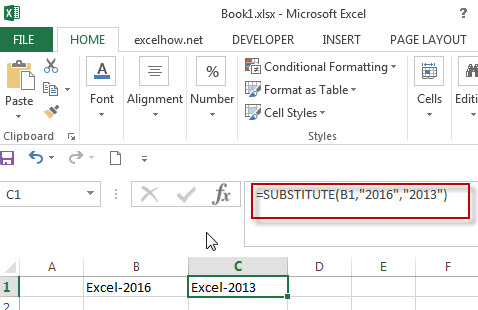In the above example, the SUBSTITUTE function is going to search the old_text in the second argument, if it found the “2016”, then replace it with new_text “2013”.

2# if you know the position of the text that you want to replace, and you can use the REPLACE function.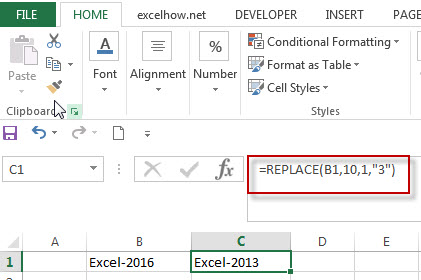### Related Functions

• Excel Replace function
The Excel DATE function returns the serial number for a date.The syntax of the DATE function is as below:= DATE (year, month, day) …
• Excel Substitute function
The Excel SUBSTITUTE function replaces a new text string for an old text string in a text string. ….

## Excel Replace Function Remove Text String

This post will teach you how to remove a text string of specified length from another text string in a cell.

## Excel Replace Function Remove Text String

If you want to remove text string from a cell in excel, you can use the REPLACE function. It can remove the text string by position in a string.

The Excel REPLACE function replaces all or part of a text string with another text string

The syntax of the REPLACE function is as below:

`= REPLACE(old_text, start_num, num_chars, new_text)`

For Example:

If you want to remove text string from a string in Cell B1, and the length of the string that you want to remove is 6, and the starting position number is 1, the new text character can use empty string. Now we can write down the REPLACE function to remove text string:

`=REPLACE(B1,1,6,"")`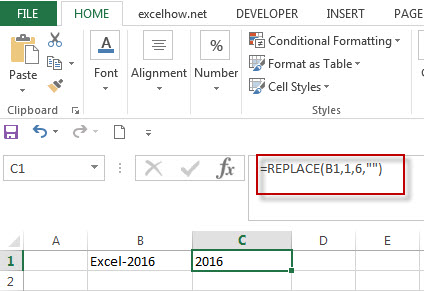### Related Functions

• Excel Replace function
The Excel DATE function returns the serial number for a date.The syntax of the DATE function is as below:= DATE (year, month, day) …

## Combining the REPLACE Function with FIND Function to Remove Text in Excel

How to remove a text string or characters from a cell when the text is at a specific position. And this post will guide you how to remove a text string from a cell or column that the removed text is at a variable position in excel.

## Combining the Replace Function with Text Function to Remove Text

You can use FIND function to get the position of the specified character within the text string, and then combining within REPLACE function to remove the text. For example:

`=FIND("-"B1)`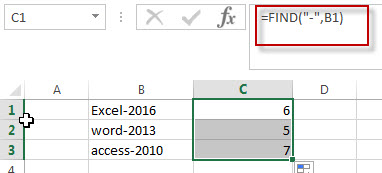In the above formula, the FIND function will return the position of hyphen character (-) from the cell B1.

The Excel REPLACE function replaces all or part of a text string with another text string

The syntax of the REPLACE function is as below:

`= REPLACE(old_text, start_num, num_chars, new_text)`

If you want to remove the characters before the hyphen character, then you need use FIND function to get the position of hyphen in the text,  then combine with the REPLACE function and then we can write down the below formula:

`=REPLACE(B1,1,FIND("-",B1),"")`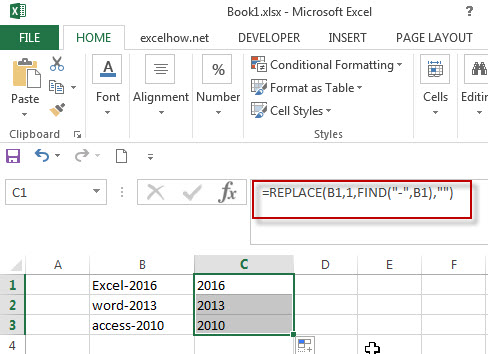To remove text, you can use an empty string as “new_text” argument in REPLACE function.

### Related Functions

• Excel Replace function
The Excel DATE function returns the serial number for a date.The syntax of the DATE function is as below:= DATE (year, month, day) …
• Excel Find function
The Excel FIND function returns the position of the first text string (substring) from the first character of the second text string. The FIND function is a build-in function in Microsoft Excel and it is categorized as a Text Function….

## Combining the Replace function with Text function in Excel

In excel, we can use Replace function in combination with Text function to replace date characters that are part of the text string. And this post will guide you how to use TEXT function as the first arguments to replace text string in REPLACE function in excel 2013.

We have talked in the previous post that the TEXT function will return a text string, so the returned result of Text function can be as the first argument in the REPLACE function.

The Excel REPLACE function replaces all or part of a text string with another text string

The syntax of the REPLACE function is as below:

`= REPLACE(old_text, start_num, num_chars, new_text)`

## Combining the Replace function with Text function

Assuming that there is a date list in Column B, and you want to replace all month name to “DEC”.  As the date is recognized as numeric value in excel, you must convert the date as text string using TEXT function.  So we can write down the following REPLACE function:

`=REPLACE(TEXT(B1,”dd-mmm-yyyy”),4,3,”DEC”)`

We can enter the above REPLACE formula into Cell B1, then drag the Fill Handle to the entire Column B. it will apply for the other cells for this formula. Then you will get the expected results. Let’s see the following screenshot: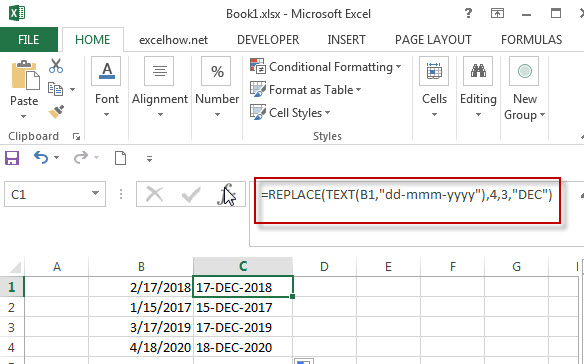### Related Functions

• Excel Replace function
The Excel REPLACE function replaces all or part of a text string with another text string.The syntax of the REPLACE function is as below:=REPLACE (old_text, start_num, num_chars, new_text)…
• Excel Text function
The Excel TEXT function converts a numeric value into text string with a specified format. The TEXT function is a build-in function in Microsoft Excel and it is categorized as a Text Function. The syntax of the TEXT function is as below: = TEXT (value, Format code)…

## Excel Replace function with numeric values

In excel, how to replace a numeric characters within a text string using another numeric characters. This post will guide you how to use REPLACE function to replace numeric characters using another numeric characters in one text string.

The Excel REPLACE function replaces all or part of a text string with another text string

The syntax of the REPLACE function is as below:

`=REPLACE(old_text, start_num, num_chars, new_text)`

If you want to replace numeric characters that are part of text string with another text string, it can be numeric characters, for example: replacing the numeric characters “2010”of text string “excel 2010” with another number “2016”, you can use the following REPLACE function in excel 2016:

`=REPLACE(B2,7,4,2016)`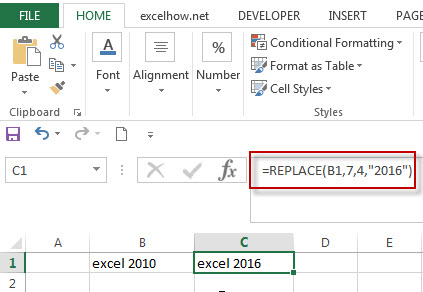The returned results of REPLACE function is still a text string, you can see that the text string “excel 2016” be returned.

You can also just replace one or more digits within a number, we can use another REPLACE formula to reflect the above request.

```=REPLACE(B1,10,1,6)
```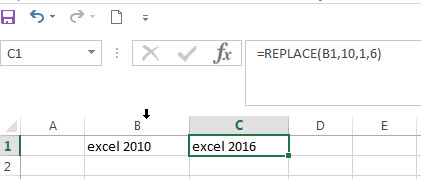In the above example, the REPLACE Function will only replace one digit that are part of numeric characters with a new numeric value “6”. The text string “excel 2016” will be returned.

### Related Functions

• Excel Replace function
The Excel DATE function returns the serial number for a date.The syntax of the DATE function is as below:= DATE (year, month, day) …

## Convert Date to Day of Week in Excel (get day name from date )

In excel, how to get the day of week from an excel date value. How to return a day name of the week from a date that is contained in a cell in Microsoft Excel. This post will guide you how to convert date to day of week only using Text Function in Microsoft Excel.

## Convert date to day of week with Text Function (get day of week from date)

If you want to get the day name from a date in excel, you can use the TEXT function with a specified format code like “ddd” or “dddd”. We can try to use the below TEXT formula in excel:

```=TEXT(date,"ddd")
=TEXT(date, "dddd")```

If you want to get the day name of the week as an abbreviation from a date, such as: Mon, Tue, etc. Enter the following TEXT formula in the Cell C1 that you want to get the day name of the week, and then press ENTER:

`=TEXT(B1,"ddd")`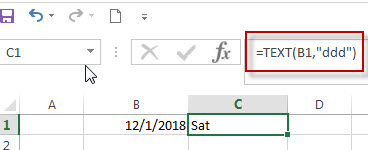If you want to get the full day name of the week, such as: Monday, Tuesday, etc. Enter the following TEXT formula in the Cell C1 that you want to get the day name of the week, then press ENTER:

`=TEXT(B1,"dddd")`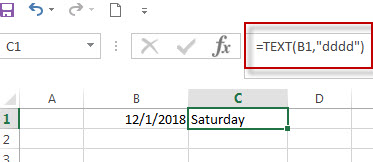## Get a Day Name of the Week Using WEEKDAY and CHOOSE function

The WEEKDAY function will get a day number of the week form a date.

We can use the returned value of WEEKDAY function as the first argument within the CHOOSE function. The CHOOSE function will use the returned weekday number as the first argument to return the nth day name from a list of day name.

So we can use the following CHOOSE function in combination with WEEKDAY function to get the full day name of the week from a date.

`=CHOOSE(WEEKDAY(B1),"Sunday","Monday","Tuesday","Wedesday","Thusday","Friday","Saturday")`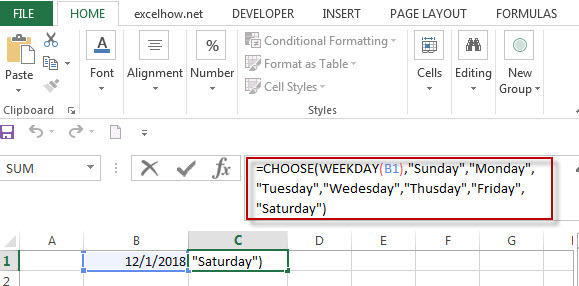For more format codes in excel formatting, you can refer to the below table:

 Format Code Description Examples 0 only display digits in its place #.00 – Forces the function to display two decimal places =Text(34.234,”\$##.00″) result: \$34.23 # Display the placeholder =Text(4.527,”#.##) result: 4.53 . the position of Decimal Point =Text(342.2,”0.00″) result: 342.20 d Day of the month or day of week d- one or two digit number (1-31) dd- two digit number (01-31) ddd-abbreviated day of week (Mon to Sun) dddd-full name of day of week(Monday to Sunnday) =Text(TODAY(),”DDDD”) result: Monday m The Month of the Year m- one or two digit number mm-two digit number mmm-abbreviated month(Jan to Dec) mmmm-full name of month(January to December)) =Text(TODAY(),”MM/DD/YY”) result:11/06/17 y year yy-two digit representation of year(e.g.01,17) yyyy-four digit representation of year(e.g. 2001,2017) =Text(TODAY(),”MM/DD/YY”) result:11/06/17

### Related Formulas

• Excel Convert numbers to Text
The Text function will accept a numeric value as the first argument, then based on the format code in the second argument to convert the number to text. You can convert all the standard number formats such as: dates, times, currency to Text string in excel.…
• Convert date to month name with Text Function
If you want to convert the date to a month name, you can use the TEXT function with a specified format code like “mmm”in excel.  You can try to run the following TEXT formula:=TEXT(B1,”mmm”)
• Convert Date to text with Text Function in Excel
you can use TEXT function in excel to convert dates to text in a specific format code. For example, The TEXT function can use the following patterns, like as: “mm/dd/yyyy”, “yyyy/mm/dd”, etc.
• Convert date to month and year only in excel
If you want to convert the date to month and year only, you can use “yyyymm” format code within the TEXT function in excel, so you can write down the below TEXT formula:=TEXT(date,”yyyymm”)
• Convert date to month and day only in excel
If you want to convert the date (mm/dd/yyyy) to month and day only, you can use “mm dd” format code within the TEXT function in excel, so you can write down the below TEXT formula: =TEXT(date,”mm dd”)

### Related Functions

• Excel Text function
The Excel TEXT function converts a numeric value into text string with a specified format. The TEXT function is a build-in function in Microsoft Excel and it is categorized as a Text Function. The syntax of the TEXT function is as below: = TEXT (value, Format code)…
• Choose function in excel
The Excel CHOOSE function returns a value from a list of values. The CHOOSE function is a build-in function in Microsoft Excel and it is categorized as a Lookup and Reference Function.The syntax of the CHOOSE function is as below:=CHOOSE (index_num, value1,[value2],…)…
• Excel WEEKDAY function
The Excel WEEKDAY function returns a integer value representing the day fo the week for a given Excel date and the value is range from 1 to 7.The syntax of the WEEKDAY function is as below:=WEEKDAY (serial_number,[return_type])…

## Convert date to month and day only in excel

In excel, how to get the month and day string from an excel date value. How to convert the dates to text string, just only display as month and day. This post will guide you how to convert date to month and day value only using Text Function in Microsoft Excel.

## Convert date to month and day with Text Function

If you want to convert the date (mm/dd/yyyy) to month and day only, you can use “mm dd” format code within the TEXT function in excel, so you can write down the below TEXT formula:

`=TEXT(date,"mm dd")`

Assuming that you want to get the text string of month and year only from the date value: 12/1/2018 in Cell B1, then you can add another column and use the below formula in Cell C1 like:

`=TEXT(B1,"mm dd")`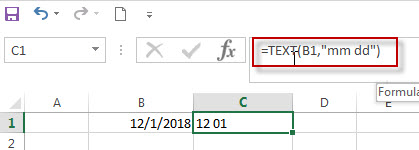If you are using the format code as “mmmm dd”, then the full name of month will display in Cell C1.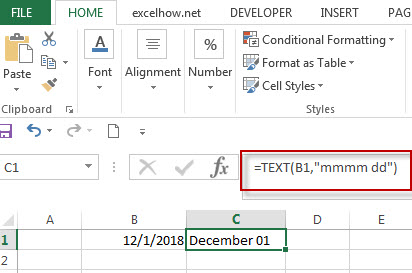For more format codes in excel formatting, you can refer to the below table:

 Format Code Description Examples 0 only display digits in its place #.00 – Forces the function to display two decimal places =Text(34.234,”\$##.00″) result: \$34.23 # Display the placeholder =Text(4.527,”#.##) result: 4.53 . the position of Decimal Point =Text(342.2,”0.00″) result: 342.20 d Day of the month or day of week d- one or two digit number (1-31) dd- two digit number (01-31) ddd-abbreviated day of week (Mon to Sun) dddd-full name of day of week(Monday to Sunnday) =Text(TODAY(),”DDDD”) result: Monday m The Month of the Year m- one or two digit number mm-two digit number mmm-abbreviated month(Jan to Dec) mmmm-full name of month(January to December)) =Text(TODAY(),”MM/DD/YY”) result:11/06/17 y year yy-two digit representation of year(e.g.01,17) yyyy-four digit representation of year(e.g. 2001,2017) =Text(TODAY(),”MM/DD/YY”) result:11/06/17

### Related Formulas

• Excel Convert numbers to Text
The Text function will accept a numeric value as the first argument, then based on the format code in the second argument to convert the number to text. You can convert all the standard number formats such as: dates, times, currency to Text string in excel.…
• Convert date to month name with Text Function
If you want to convert the date to a month name, you can use the TEXT function with a specified format code like “mmm”in excel.  You can try to run the following TEXT formula:=TEXT(B1,”mmm”)
• Convert Date to text with Text Function in Excel
you can use TEXT function in excel to convert dates to text in a specific format code. For example, The TEXT function can use the following patterns, like as: “mm/dd/yyyy”, “yyyy/mm/dd”, etc.
• Convert date to month and year only in excel
If you want to convert the date to month and year only, you can use “yyyymm” format code within the TEXT function in excel, so you can write down the below TEXT formula:=TEXT(date,”yyyymm”)

### Related Functions

• Excel Text function
The Excel TEXT function converts a numeric value into text string with a specified format. The TEXT function is a build-in function in Microsoft Excel and it is categorized as a Text Function. The syntax of the TEXT function is as below: = TEXT (value, Format code)…

## Three Ways to Convert Number to Text in Excel

Before we have wrote a post to describe that how to convert number to Text string with Text Function in excel. This post will guide you how to convert number to Text using The Format Cells Command and Text to Columns wizard in excel.

## Convert Number to Text Using Format Cells Option

You also can be used the Format Cells option to change the number to Text string in excel.  It may be another quick way to change the numeric value to text string in excel.

1# select the range with the numbers that you want to convert to text.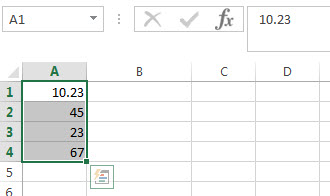2#Right-click the selected range in the step1, and then click “Format Cells...” item from the pop-up menu list.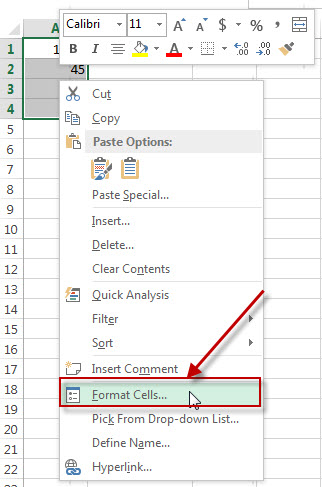3# Format Cells” window will appear.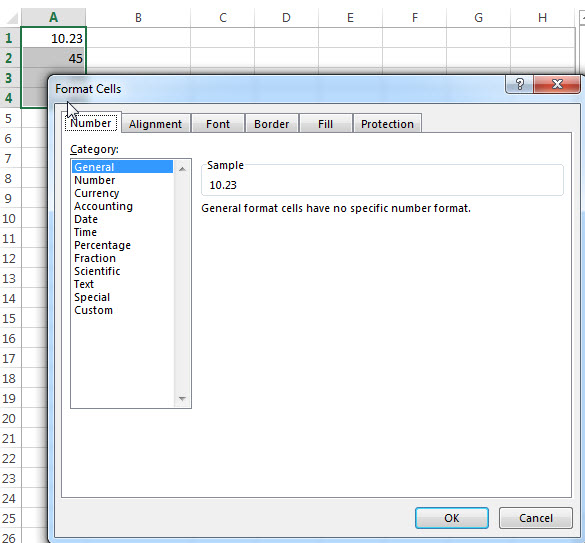4# Select “Text” under the Number tab and then click OK button.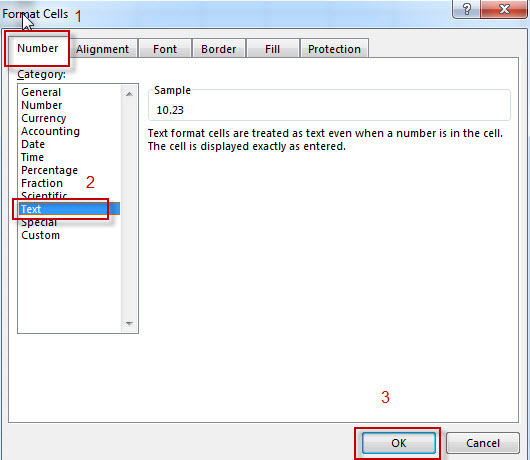## Convert Number to Text Using Text to Columns wizard

1# Select the column that you want to convert numbers to text string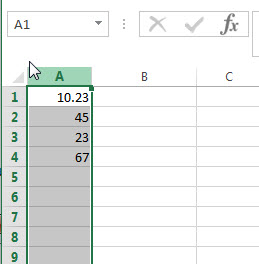2# click on the “Text to Columns” button under DATA tab on excel ribbon.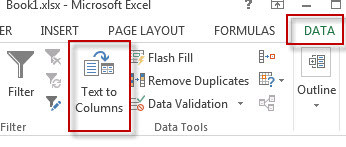3#Convert Text to Columns Wizard” window will appear.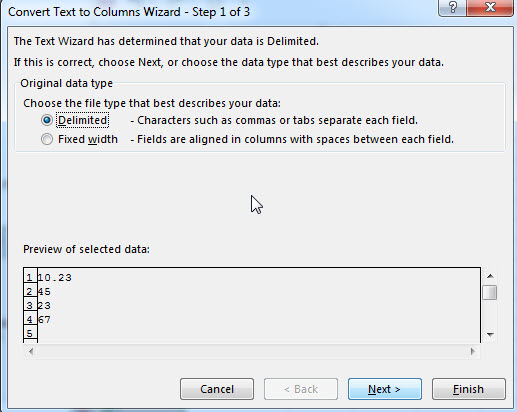4# click “next” button through step 1 and step 2. Select “Text” radio button under step 3. Then click on “Finish” button.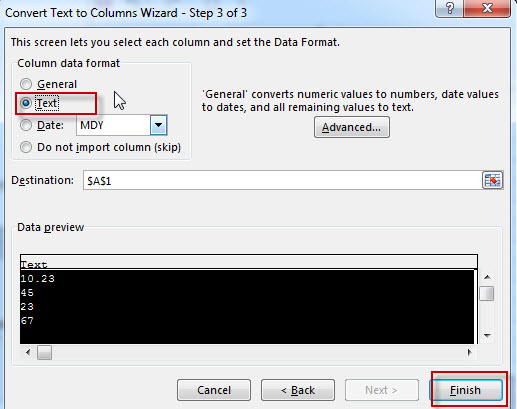You will see that the numbers is converted to text now.

## Convert Number to Text Using Concatenate Operator

We can also use another way to change the numeric value to string using Excel Concatenate operator.

The concatenation operator (&) can join together text and numbers into a single text string in excel. So if you just concatenate an empty text string (such as: “”) and a numeric value together, the returned value will be a text string. Let’s see the below example:

`=""&A1`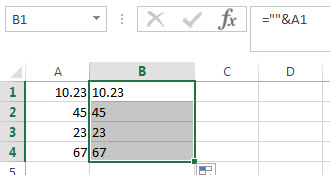You need to enter the above excel formula in the B1 cell, then click “enter”. You will see that the numeric value in Cell A1 be changed as text string in B1.

Also read: Excel Convert numbers to Text

## Excel Convert numbers to Text

This post will guide you how to convert numbers to text with the Excel TEXT function. Assuming that you want to display leading Zero in numbers in cell, then you need to convert them into Text based on the specified Format_text. So you can use TEXT function to achieve this in Excel.

The Text function will accept a numeric value as the first argument, then based on the format code in the second argument to convert the number to text. You can convert all the standard number formats such as: dates, times, currency to Text string in excel.

## Convert Number to Text with Text Function

If you just want to convert the numbers in the cell to Text without any formatting, then you can use the below generic formula of Text function in excel:

`=Text(B1,"0")`

​In the above formula, it will convert the number to text string with no special formatting, as we just use the most simply format text as “0“.

If you want to display the leading zero in numbers, then you can use the format_text as “0000” in the Excel Text function as follows:

`=Text(B1,"0000")`

If you want to convert the number in cell B1 to text string which keep three decimal digits, the following format text can be used in the Excel Text function:

00.000

we can write down the formula using Text function in excel as follows:

`=Text(B1,"00.000")`

For more format codes in excel formatting, you can refer to the below table:

 Format Code Description Examples 0 only display digits in its place #.00 – Forces the function to display two decimal places =Text(34.234,”\$##.00″) result: \$34.23 # Display the placeholder =Text(4.527,”#.##) result: 4.53 . the position of Decimal Point =Text(342.2,”0.00″) result: 342.20 d Day of the month or day of week d- one or two digit number (1-31) dd- two digit number (01-31) ddd-abbreviated day of week (Mon to Sun) dddd-full name of day of week(Monday to Sunnday) =Text(TODAY(),”DDDD”) result: Monday m The Month of the Year m- one or two digit number mm-two digit number mmm-abbreviated month(Jan to Dec) mmmm-full name of month(January to December)) =Text(TODAY(),”MM/DD/YY”) result:11/06/17 y year yy-two digit representation of year(e.g.01,17) yyyy-four digit representation of year(e.g. 2001,2017) =Text(TODAY(),”MM/DD/YY”) result:11/06/17 h Hour h-one or two digit number (e.g. 1,23) hh-two digit number (e.g. 01,23) =Text(14:16,”hh:mm”) result: 14:16 m Minute m-one or two digit representation (e.g. 1,59) mm-two digit representation (e.g. 01,59) =Text(14:16,”hh:mm”) result: 14:16 s Second s-one or two digit representation (e.g. 1,59) ss=two digit representation (e.g. 01,59)

### Related Functions

• Excel Text function
The Excel TEXT function converts a numeric value into text string with a specified format. The TEXT function is a build-in function in Microsoft Excel and it is categorized as a Text Function. The syntax of the TEXT function is as below: = TEXT (value, Format code)…